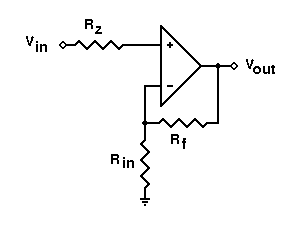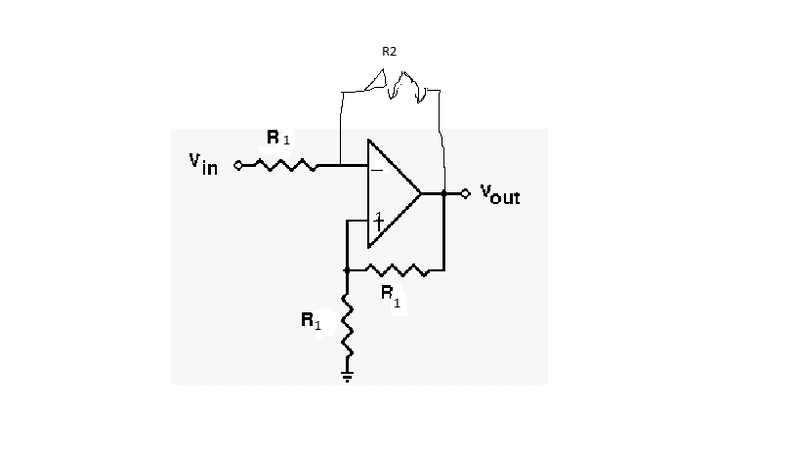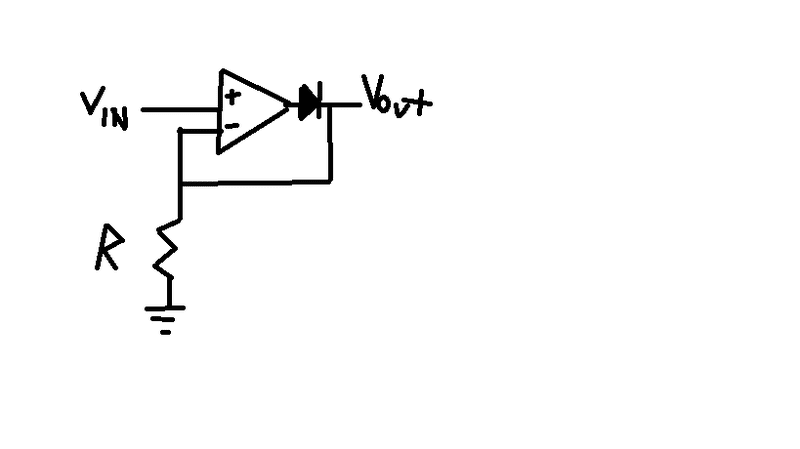# Op-Amp Circuit ANalysis

• Engineering
• thercias

## Homework Statement

Consider the circuit drawn belowThis diagram was the closest I could find online to the one equivalent to my question, but there are some adjustments I need to clarify.
1)Replace Rz, Rf, and Rin, to R1.
2)Also, in my question, there's an extra wire connecting Vout to the right of Rz. In this wire, there is a resistor in the middle, called R2.
3) Switch the + symbol to the negative side and the - symbol to the + side.

Edit: Here I redrew it in paint to give a better illustration of the circuit:Now for the question:
A) In terms of Vin, Vout, R1, and R2, what is the voltage at the non-inverting input to the op-amp (v+)?
B) In terms of Vin, Vout, R1, and R2, what is the input current to the circuit ( the current through R1)?
c) In terms of Vin, R1, and R2, what is the output voltage (Vout) of the circuit.

## Homework Equations

Kirchoff's loop equations
V+ = V-
V= IR

## The Attempt at a Solution

I guess something I'm confused about in this question is if this is considered to be a non-inverting or inverting amplifer, since the wires go from both sides of Vout.

A)
So one of the main rules for op amps is that the voltage at the non inverting input will equal the voltage of the inverting input. This can be solved by using a voltage divider.
Therefore, at Voltage at the non inverting input, V+ = Vout(R1/(R1+R1)) = Vout/2
Also V+ = V- = Vin can be another way to express this. Therefore we also get the expression Vin = Vout/2

However, I'm not sure if this is correct, since the question wanted it in terms of 4 variables, while I only have it in terms of Vout.

B)
current = i = (V(-) - Vin)/R1 =[Vin-Vin]/R1
So the current cancels and becomes 0, which doesn't make sense. So something is wrong with part A.

I'm having trouble finding the voltage through the non inverting input for starters, so if anyone can help me through that it would be appreciated.

Last edited:

Also V+ = V- = Vin can be another way to express this. Therefore we also get the expression Vin = Vout/2

There's no reason why V- is equal to Vin.There's a current going through the R1 connected to Vin, so you get a voltage drop.

Ok so I redid that bit.

So we have V(-) = Vout/2

Using kirchhoffs law, the current through the R1 would be

Vin - IR1 - Vout/2 = 0
therefore, I = (Vin - 0.5Vout)/R1

and Vout would be

-Vout/2 - IR2 = Vout
Vout = IR2/1.5
=-R2(Vin-0.5Vout)/1.5R1

Would this be correct? I'm kind of confused when or not to use a positive or negative sign when making kirchhoffs equation for Vout/2

## The Attempt at a Solution

I guess something I'm confused about in this question is if this is considered to be a non-inverting or inverting amplifer, since the wires go from both sides of Vout.

where is the signal being fed into ? the - input so that tells you that the circuit is an _______
you fill in the blank

where is the signal being fed into ? the - input so that tells you that the circuit is an _______
you fill in the blank
It would be an inverting amplifier. So, the most difficult part for me in understanding these circuits is coming up with an expression for V+ or V-. For some reason I'm just not grasping some fundamental concept,no matter how hard I try and research. If R1 is grounded, how would that affect V+? (I understand I already solved for V+, but still trying to understand this conceptually).

Also, how does my above solution look.

you should have labelled your resistors differently
having 3 x R1 's is somewhat confusing when you ask Q's or someone tries to explain

for a moment ( partly because I have never seen 2 feedback resistors --- a little unsure how to attack that ;) )
lets ignore the R1 feedback resistor to from output to +in

if +in is grounded, then the op-amp will follow the rule that it will do what it can to make -in = +in so if +in = 0V, -in will = 0V ( when its in closed loop mode ie. there is feedback)

current I, through the input R1 = Vin / (input)R1

now remember the other op-amp rule ... NO current flows in or out of either input

so where is the current flowing ? through input R1 and ______

Last edited:
Current is flowing through R1 and R2. But they are separated by a junction. So would I = Vin/R1 = Vout/R2 ?

And V(out) = V(-) + IR2?

edit: I wouldn't be Vout/R2, it would be V2/R2

Last edited:
Current is flowing through R1 and R2. But they are separated by a junction. So would I = Vin/R1 = Vout/R2 ?

yes but treat each part separately

initially you work out the current through input R1= Vin / (input)R1
that current cannot flow into the -in input of the op-amp so it flows up and through R2
That will produce a voltage drop across R2 = ______

you are maybe seeing that this can all be done with Ohms law :)

Have you learn about input bias current of an op-amp yet ?
if so we can then explain the resistor from +in to ground

Last edited:
Voltage drop across R2 = IR2 = VinR2/R1
So Vout would be = Vout/2 + VinR2/R1
Vout =2VinR2/R1 ?

Is the input bias current difference through the current from the + and - inputs?

As for explaining ground, I think this example is kind of hard to understand since the R1s get in the way. For something like this,The resistor is being grounded. Does that mean the voltage through the - input simply becomes the output voltage? And would the voltage across the resistor also be Voutput then? And the voltage through the diode be v input?

Last edited:
lets put some practical values in here ( I work easier that way )

Vin=1V, R1 = 1k, R2 = 10k

so I through R1 = 1V/1k = 1mA
V drop across R2 = I x R2 = 1mA x 10k = 10V
The R1, R2, -in node is going to be positive relative to the R2, op-amp output pin
so we know that the R1, R2, -in node is 0V, that means the output of the op-amp is going to be -10V ( relative to the input)

Input bias current ...

as per the rule ( Ideal op-amp) No current flows in or out of the op-amp inputs
in reality, ALL op-amps have a very small input bias current --- nano to micro amps
This results in an input offset voltage ( a voltage error) between the input pins.

the input bias current applies equally to both inputs. This is the reason for the resistor connecting the "+" input to ground. If this resistor is close in value to the parallel combination of R1 and R2 the same voltage error will be generated at the two inputs, and will therefore be canceled out, or very nearly to the point of making it unimportant..

For many general applications, the input bias current is so small that any offset voltage error can be ignored. Its only when you are dealing with high gain and low noise applications ( eg preamplifiers) that you want to eliminate that error voltage as it only adds to the noise figure of the circuit

Dave

Unfortunately, I'm not really understanding. So if my answers for part A) and B) are right, I don't understand what is going wrong with part C).
Current through R1 = Vin/R1. Voltage drop across R2 = IR2. Therefore the voltage drop across R2 = Vin*R2/R1

I also thought, applying kirchhoffs rule, V(-) + IR2 = V(out).

Now all that discussion should cover everything EXCEPT the additional feedback resistor to the +in pin

As I said earlier, I haven't come across that example and not sure how to help explaining that
hopefully some one else will chime in :)

D

Therefore the voltage drop across R2 = Vin*R2/R1

yes, but more simply ...

Voltage drop across R2 = IR2

Vout =2VinR2/R1 ?

no ... if you plug in the values I used as an example you will see it gives the wrong answer ( twice the value ... 20V instead of 10V)

now remember what I said a couple of posts ago ...

The R1, R2, -in node is going to be positive relative to the R2, op-amp output pin node
so we know that the R1, R2, -in node is 0V, that means the output of the op-amp is going to be -10V ( relative to the input)

so gain ( because its an inverting amplifier) = -R2 / R1 = -10000 / 1000 = -10

therefore ... + 1V in = -10V out, -1V in = +10V out

Last edited:
time for bed for me here in Australia
will check in tomorrow morn :)

Okay got it I think. I was getting jumbled up with the original question when writing down my answers. Vout = -VinR2/R1 in that case I think.
Thanks for the explanations, and have a good night's sleep.

Like you pointed out, I would appreciate if someone would chime in and verify if these answers are correct, or how I can work through them if they are incorrect:

A) V- = Vout/2
B)IR1 = Vin - V-
I = (Vin - V-)/R1
= (Vin - 0.5Vout)/R1

C)V(-) + IR2 = Vout
0.5Vout + R2(Vin-0.5Vout)/R1 = Vout
0.5Vout = R2(Vin - 0.5Vout)/R1
0.5Vout(1+R2/R1) = Vin*R2/R1
Vout = 2Vin*R2/R1/(1+(R2/R1))
=2*R2*Vin/(R1+R2)

These are indeed all correct